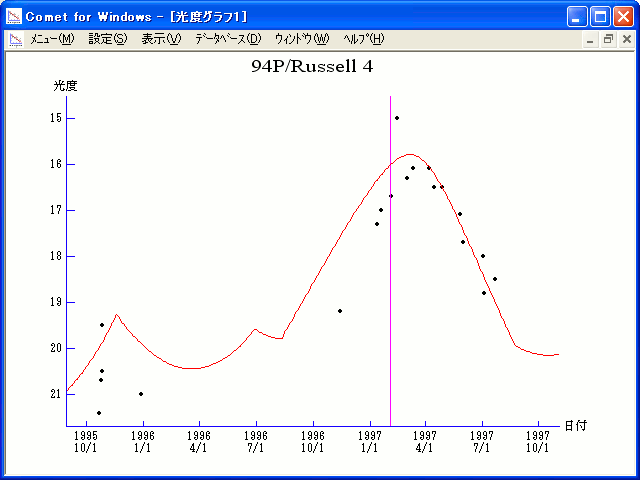# \$B%i%C%;%kBh#4WB@1(B

94P/Russell 4 (1997)###\$B50F;MWAG(B

```   The following improved orbital elements by Kenji Muraoka, are
from 119 observations 1984 to 1997, perturbations by 9 Plantes,
Moon and 5 minor planets were taken into account.
The mean residual is +/- 0.74 arc seconds.

Epoch  =  1997 Feb.  1.0  TT       JDT = 2450480.5
T  =  1997 Feb.  3.49811       +/- 0.00050 (m.e.) TT
Peri. =   93.27034                +/- 0.00025
Node  =   70.96930                +/- 0.00023   (2000.0)
Incl. =    6.18812                +/- 0.00002
q  =    2.2292784              +/- 0.0000023 AU
e  =    0.3650973              +/- 0.0000006
a  =    3.5112129              +/- 0.0000014 AU
n  =    0.14980225             +/- 0.00000009
P  =    6.579                  +/- 0.0000038  years
```

###\$B@1?^(B1995\$BG/(B10\$B7n(B10\$BF|!A(B1996\$BG/(B11\$B7n(B 3\$BF|(B1996\$BG/(B10\$B7n(B 4\$BF|!A(B1997\$BG/(B12\$B7n(B28\$BF|(B

###\$B8wEYJQ2=(B

```        H = 14.5  G = 0.15             [    ,-175]  (              \$B!A(B1996\$BG/(B 8\$B7n(B12\$BF|(B)
m1 = 3.0 + 5 log\$B&\$(B + 35 log r  [-175, 200]  (1996\$BG/(B 8\$B7n(B12\$BF|!A(B1997\$BG/(B 8\$B7n(B22\$BF|(B)
H = 14.5  G = 0.15             [ 200,    ]  (1997\$BG/(B 8\$B7n(B22\$BF|!A(B              )
```##### \$B50F;MWAG\$OB<2,7r<#;a\$N7W;;\$K\$h\$k\$b\$N\$G\$9!#(B \$B@1?^\$O(B StellaNavigator Ver.2.0 for Windows (\$B%"%9%H%m%"!<%D(B \$BJTCx(B / \$B%"%9%-!<=PHG6I4)(B) \$B\$G:n@.\$7\$?\$b\$N\$G\$9!#(B \$B8wEY%0%i%U\$O(BComet for Windows\$B\$G:n@.\$7\$?\$b\$N\$G\$9!#(B